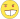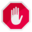## Name

ST_Intersection — Computes a geometry representing the shared portion of geometries A and B.

## Synopsis

`geometry ST_Intersection(` geometry geomA , geometry geomB , float8 gridSize = -1 `)`;

`geography ST_Intersection(` geography geogA , geography geogB `)`;

## Description

Returns a geometry representing the point-set intersection of two geometries. In other words, that portion of geometry A and geometry B that is shared between the two geometries.

If the geometries have no points in common (i.e. are disjoint) then an empty atomic geometry of appropriate type is returned.

If the optional `gridSize` argument is provided, the inputs are snapped to a grid of the given size, and the result vertices are computed on that same grid. (Requires GEOS-3.9.0 or higher)

ST_Intersection in conjunction with ST_Intersects is useful for clipping geometries such as in bounding box, buffer, or region queries where you only require the portion of a geometry that is inside a country or region of interest.For geography this is a thin wrapper around the geometry implementation. It first determines the best SRID that fits the bounding box of the 2 geography objects (if geography objects are within one half zone UTM but not same UTM will pick one of those) (favoring UTM or Lambert Azimuthal Equal Area (LAEA) north/south pole, and falling back on mercator in worst case scenario) and then intersection in that best fit planar spatial ref and retransforms back to WGS84 geography.This function will drop the M coordinate values if present.If working with 3D geometries, you may want to use SFGCAL based ST_3DIntersection which does a proper 3D intersection for 3D geometries. Although this function works with Z-coordinate, it does an averaging of Z-Coordinate.

Performed by the GEOS module

Enhanced: 3.1.0 accept a gridSize parameter

Requires GEOS >= 3.9.0 to use the gridSize parameter

Changed: 3.0.0 does not depend on SFCGAL.

Availability: 1.5 support for geography data type was introduced.This method implements the OGC Simple Features Implementation Specification for SQL 1.1.

s2.1.1.3This method implements the SQL/MM specification.

SQL-MM 3: 5.1.18This function supports 3d and will not drop the z-index.

However, the result is computed using XY only. The result Z values are copied, averaged or interpolated.

## Examples

```SELECT ST_AsText(ST_Intersection('POINT(0 0)'::geometry, 'LINESTRING ( 2 0, 0 2 )'::geometry));
st_astext
---------------
GEOMETRYCOLLECTION EMPTY

SELECT ST_AsText(ST_Intersection('POINT(0 0)'::geometry, 'LINESTRING ( 0 0, 0 2 )'::geometry));
st_astext
---------------
POINT(0 0)```

Clip all lines (trails) by country. Here we assume country geom are POLYGON or MULTIPOLYGONS. NOTE: we are only keeping intersections that result in a LINESTRING or MULTILINESTRING because we don't care about trails that just share a point. The dump is needed to expand a geometry collection into individual single MULT* parts. The below is fairly generic and will work for polys, etc. by just changing the where clause.

```select clipped.gid, clipped.f_name, clipped_geom
from (
select trails.gid, trails.f_name,
(ST_Dump(ST_Intersection(country.geom, trails.geom))).geom clipped_geom
from country
inner join trails on ST_Intersects(country.geom, trails.geom)
) as clipped
where ST_Dimension(clipped.clipped_geom) = 1;```

For polys e.g. polygon landmarks, you can also use the sometimes faster hack that buffering anything by 0.0 except a polygon results in an empty geometry collection. (So a geometry collection containing polys, lines and points buffered by 0.0 would only leave the polygons and dissolve the collection shell.)

```select poly.gid,
ST_Multi(
ST_Buffer(
ST_Intersection(country.geom, poly.geom),
0.0
)
) clipped_geom
from country
inner join poly on ST_Intersects(country.geom, poly.geom)
where not ST_IsEmpty(ST_Buffer(ST_Intersection(country.geom, poly.geom), 0.0));```

## Examples: 2.5Dish

Note this is not a true intersection, compare to the same example using ST_3DIntersection.

```select ST_AsText(ST_Intersection(linestring, polygon)) As wkt
from  ST_GeomFromText('LINESTRING Z (2 2 6,1.5 1.5 7,1 1 8,0.5 0.5 8,0 0 10)') AS linestring
CROSS JOIN ST_GeomFromText('POLYGON((0 0 8, 0 1 8, 1 1 8, 1 0 8, 0 0 8))') AS polygon;

st_astext
---------------------------------------
LINESTRING Z (1 1 8,0.5 0.5 8,0 0 10)
```